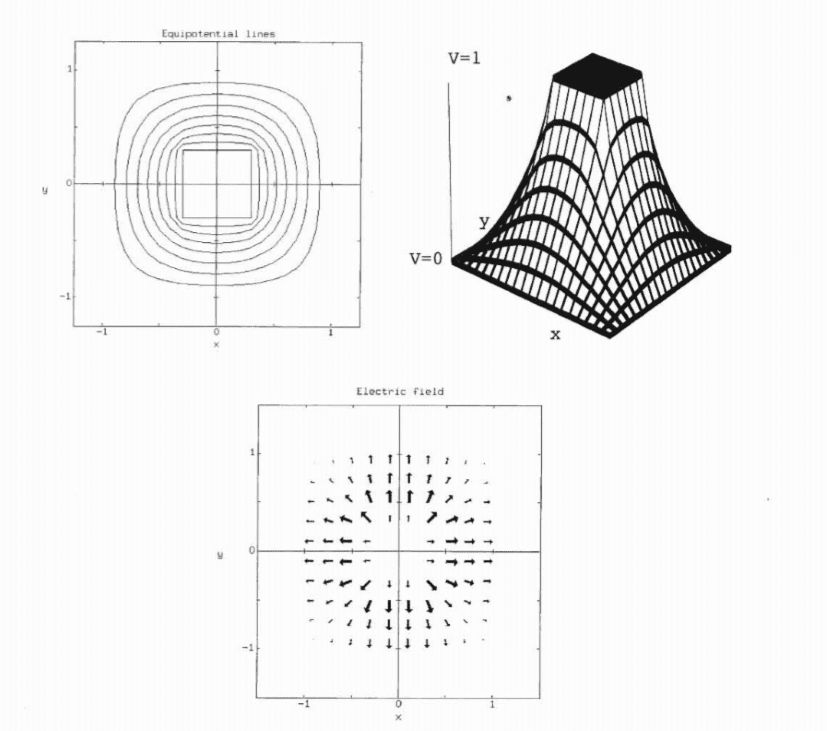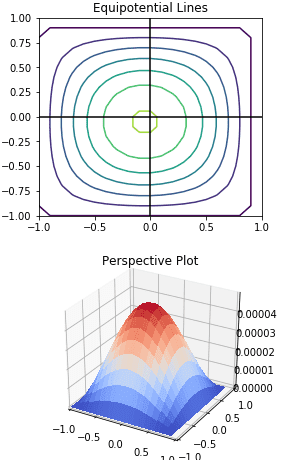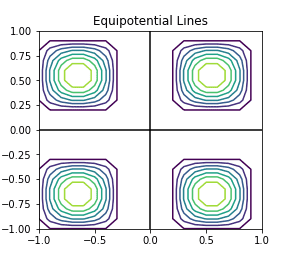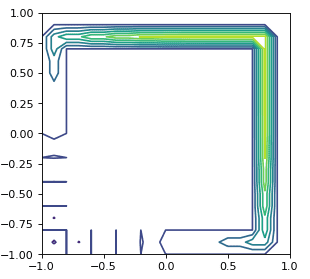# Trouble with Electric Potential Boundaries (Computational Physics)

CrosisBH
Homework Statement:
Recreate the given figure. A block (I don't know what width I'm eyeballing it) of constant potential 1 is centered at the origin, use the Jacobi method to find the potential around the block (and eventually the E-field)
Relevant Equations:
$$\Delta V_{end} \leq 10^{-5}$$, meaning the program stops when this is satisfied
$$V_{n+1}(i,j) = \frac{1}{4} [V_n(i-1,j)+V_n(i+1,j)+V_n(i,j-1)+V_n(i,j+1)]$$This is in python:
Python:
#ELECTRIC POTENTIAL

from mpl_toolkits.mplot3d import Axes3D
from matplotlib import cm
import numpy as np
import matplotlib.pyplot as plt

dx = 0.1
dy = 0.1

xrange=np.arange(-1,1,dx)
yrange=np.arange(-1,1,dy)

X,Y = np.meshgrid(xrange, yrange)

max_dV = 10e-5

V = np.zeros((int(2/dx),int(2/dy)))
V[i+int(len(V)/2)][j+int(len(V)/2)] = 1

#setting up the boundries for guessing
for i in range(0,len(V)):
for j in range(0,len(V)):
if(i==0):
V[i][j] = 1
if(i==len(V)-1):
V[i][j] = 1
if(j==0):
V[i][j] = 1
if(j==len(V)-1):
V[i][j] = 1

#Step function
def update_V(iV):
dV=0
newV=np.zeros((int(2/dx),int(2/dy)))

midX = int(len(iV)/2)
midY = int(len(iV)/2)

for i in range(1,len(iV)-1):
for j in range(1,len(iV)-1):
newV[i][j]= (iV[i-1][j]+iV[i+1][j]+iV[i][j-1]+iV[i][j+1])*0.25
dV += abs(iV[i][j] - newV[i][j])
return newV, dV

#initial plot so i can see what the hell is going on
fig = plt.figure(figsize=plt.figaspect(2.))

ax.plot([-1,1],[0,0], color = 'black')
ax.plot([0,0],[-1,1], color = 'black')
ax.contour(X,Y,V)
ax.set_title("Equipotential Lines (Before Jacobi Method)")
ax.set_xlim(-1,1)
ax.set_ylim(-1,1)

dV = 1
i=0

while(dV>max_dV):
i+=1
V, dV = update_V(V)

print("Took ", i, " steps")

fig = plt.figure(figsize=plt.figaspect(2.))
ax.plot([-1,1],[0,0], color = 'black')
ax.plot([0,0],[-1,1], color = 'black')
ax.contour(X,Y,V)
ax.set_title("Equipotential Lines")
ax.set_xlim(-1,1)
ax.set_ylim(-1,1)

ax = fig.add_subplot(2, 1, 2, projection='3d')
ax.set_title("Perspective Plot")

surf = ax.plot_surface(X, Y, V, cmap=cm.coolwarm,
linewidth=4, antialiased=False, rstride=1, cstride=1,)

plt.xlim(-1,1)
plt.ylim(-1,1)

plt.show()(Obviously there's a center issue I'm working on at the moment). This is the plot I generate. I know what the problem is, but I don't know how to handle it. The problem states that the block is a at a constant voltage, so it doesn't dissipate like this, so the center needs to stay the same. I tried treating the block as a boundary so it doesn't mess with the voltage in there, but only affects the voltage outside the block. My first try was checking if it would be updating in the region in the box by:

before doing the calculation in update_V. This produced:Interesting but not correct

I tried another (bad) approach. Where I separated it into 4 regions around the box and used 4 for loops. This produced this beautiful hot mess:This damn box is going to be the end of me. What would be a good way to approach this boundary problem?